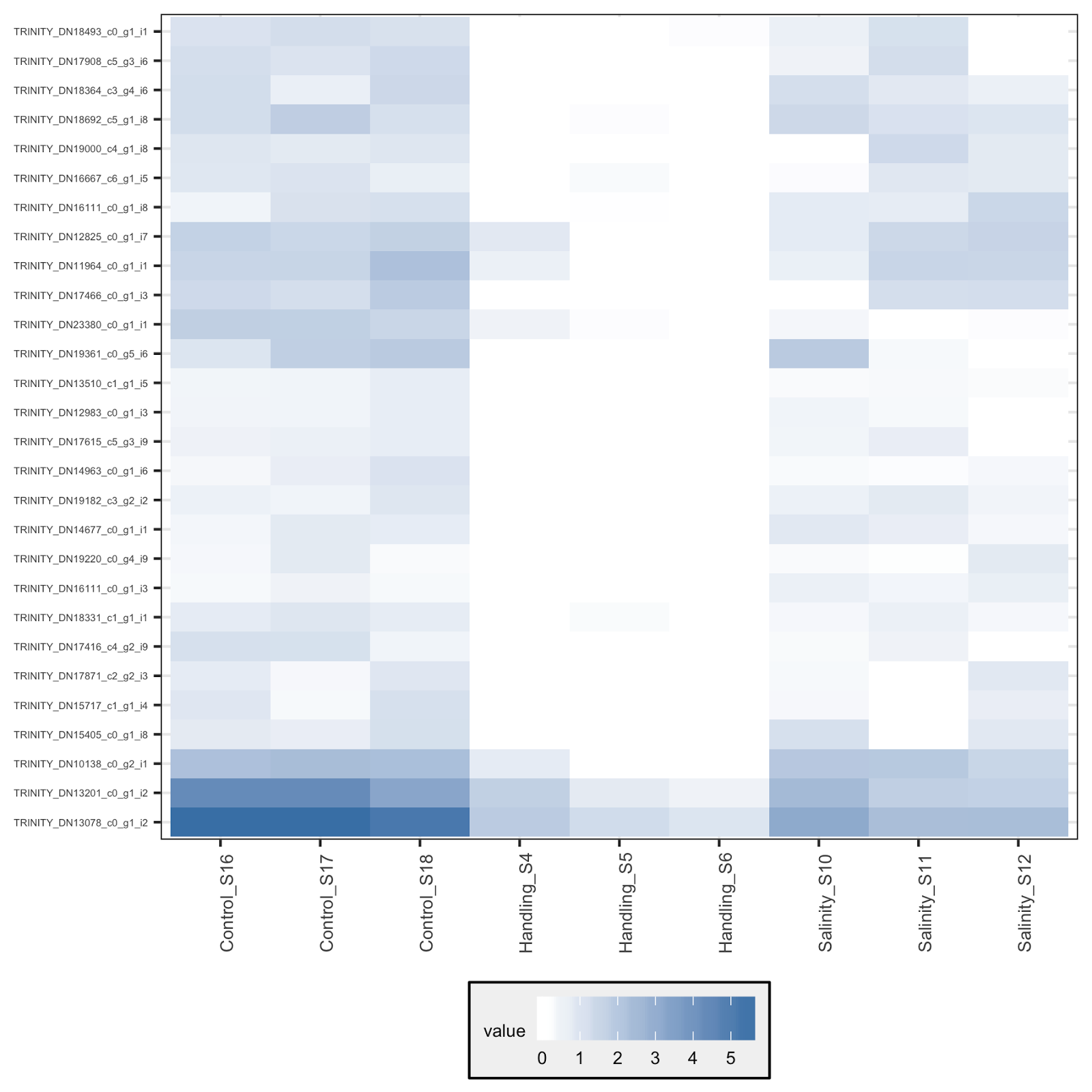Modifications of graphs from Sleuth Object?
0
0
Entering edit mode
5.9 years ago
BioBing ▴ 150

Dear Biostar users,

I have been using Kallisto/Sleuth to analyze my RNA-seq data and have a couple of questions related to the modification of heat maps based on a sleuth object (so), that I hope someone can help me with:

Question 1: I am a bit puzzled about what the "value" that goes from 0-6ish represents (see below) - what exactly does that mean? Is there a way to further modify the R-code below in order to get up- and down-regulation (minus - plus values) represented instead?

Question 2: How to modify the R-code further to add a dendrogram to the heatmap?

Thanks, Birgitte

The modified heat-map R-code:

plot_transcript_heatmap(so,Handling_type3\$target_id,units = "tpm",trans = "log", offset = 1) +
theme(axis.text.y=element_text(size=4),
axis.text.x = element_text(size=7),
legend.position = "bottom",
legend.text = element_text(size=7),
legend.title = element_text(size=7))


The resulting plot:R RNA-Seq rna-seq • 2.5k views